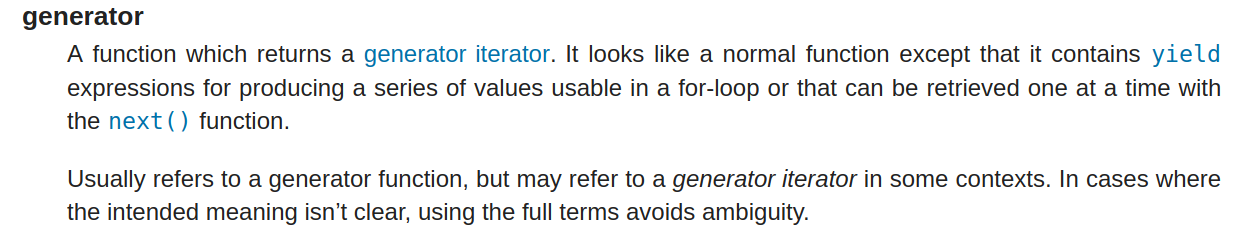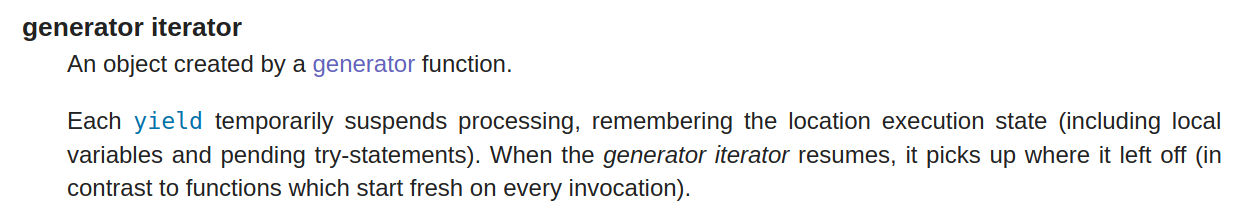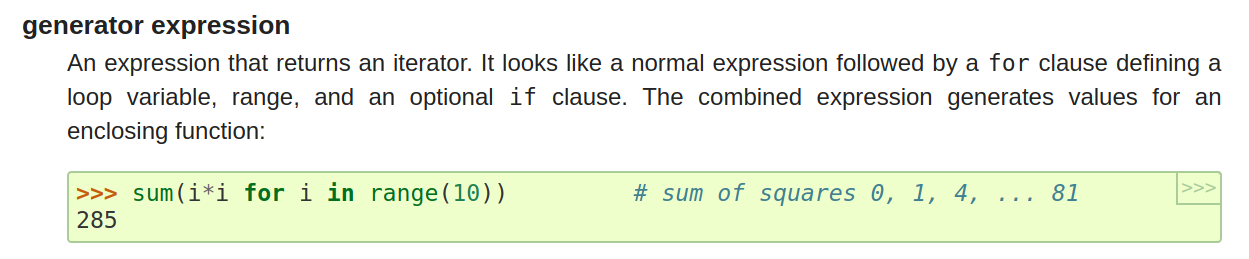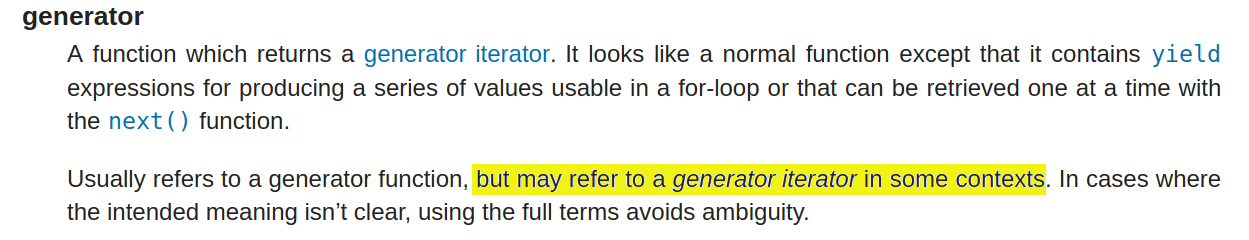# Lazy Looping

## The Next Iteration## Problem

### Find logged errors (with context)

``````
with open('logs.txt') as log_file:
prev = line = None
for next in log_file:
next = next.rstrip('\n')
if line and 'error' in line.lower():
print(prev, line, next, sep='\n')
prev, line = line, next
if line and 'error' in line.lower():
print(prev, line, None, sep='\n')
``````

## Lazy Looping

### Iterable

An iterable is anything you can loop over.

``````
for thing in my_iterable:
do_something_with(thing)
``````

### Iterator

The thing that powers iterables

A special kind of "lazy" iterable

# Loop Better

### Files are iterators

``````
>>> my_file = open("my_file.txt")
>>> next(my_file)
'This is line 1 of the file'
>>> next(my_file)
'This is line 2 of the file'
>>> for line in my_file:
...     print(line, end="")
...
This is line 3 of the file
This is line 4 (the end of the file)
>>> list(my_file)
[]
``````

### Iterators are lazy

• They compute their next value as you loop over them
• They might not store any values "inside" themselves at all

## How do you create iterators?

``````
def denumerate(iterable):
n = -1
values = []
for item in iterable:
values.append((n, item))
n -= 1
return values
``````
``````
>>> colors = ['pink', 'green', 'purple', 'blue']
>>> for n, color in denumerate(colors):
...     print(n, color)
...
-1 pink
-2 green
-3 purple
-4 blue
``````
``````
def denumerate(iterable):
n = -1
values = []
for item in iterable:
values.append((n, item))
n -= 1
return values
``````
``````
def denumerate(iterable):
n = -1

for item in iterable:
yield (n, item)
n -= 1

``````

### Generator function

``````
def denumerate(iterable):
n = -1
for item in iterable:
yield (n, item)
n -= 1
``````
``````
>>> colors = ['pink', 'green', 'purple', 'blue']
>>> items = denumerate(colors)
>>> next(items)
(-1, 'pink')
>>> list(e)
[(-2, 'green'), (-3, 'purple'), (-4, 'blue')]
>>> list(e)
[]
``````
``````
def gimme_five():
print('Start!')
return 5

def denumerate(iterable):
print('Start?')
n = -1
for item in iterable:
yield (n, item)
n -= 1

``````
``````
>>> x = gimme_five()
Start!
>>> x
5
>>> y = denumerate(["purple", "blue", "pink"])
>>> y
<generator object denumerate at 0x7febbdeaae58>
``````
``````
def denumerate(iterable):
print('start!')
n = -1
for item in iterable:
yield (n, item)
print('incrementing!')
n -= 1
print('all done!')
``````
``````
>>> for n, color in denumerate(["purple", "pink"]):
...     print(f"Color {n} is {color}")
...
start!
Color -1 is purple
incrementing
Color -2 is pink
incrementing
all done!
``````

#### Generators (and iterators) do work as you loop over them

When asked for their next item:
• They do work to figure out that item
• Yield that item to the loop they're in
• And put themselves on pause until asked for another item

``````
>>> def square_all(numbers):
...     for n in numbers:
...         yield n**2
...
>>> numbers = [2, 1, 3, 4, 7, 11]
>>> squares = square_all(numbers)
>>> squares
<generator object square_all at 0x7f11191b78b8>
>>> squares = (n**2 for n in numbers)
>>> squares_list = [n**2 for n in numbers]
>>> squares_list
[4, 1, 9, 16, 49, 121]
>>> squares
<generator object <genexpr> at 0x7f78f87af0c0>
``````

### How to make iterators

1. Write a generator function (calling it returns an iterator)
2. Make a generator expression (which makes an iterator)
3. Make an iterator class

## How are iterators used?

### Looping over iterators

``````
squares = (n**2 for n in range(1000))
total = 0
for n in squares:
total += n
``````
``````
total = sum((n**2 for n in range(1000)))
``````
``````
total = sum(n**2 for n in range(1000))
``````

### Wrapping iterators in iterators

``````
import csv
with open('expenses.csv') as expenses_file:
travel_costs = sum((
float(cost)
for date, merchant, cost, category in expense_rows
if category == 'travel'
))
``````## What do you do with iterators?

1. Wrap another iterator around them by
• passing them to a generator function
• creating a generator expression
• calling another iterator-returning function
2. Loop over them, but only once
• writing a `for` loop or a list comprehension
• calling another function that will do the looping

## The Problem: Revisited

### Find logged errors (with context)

``````
with open('logs.txt') as log_file:
prev = line = None
for next in log_file:
next = next.rstrip('\n')
if line and 'error' in line.lower():
print(prev, line, next, sep='\n')
prev, line = line, next
if line and 'error' in line.lower():
print(prev, line, None, sep='\n')
``````

### Find logged errors (with context)

``````
with open('logs.txt') as log_file:
for prev, line, next in around(strip_newlines(log_file)):
if 'error' in line.lower():
print(prev, line, next, sep='\n')
``````
``````
def around(iterable):
"""Yield (prev, item, next) for each item in iterable."""
before = current = None
for after in iterable:
if current is not None:
yield (before, current, after)
before, current = current, after
if current is not None:
yield (before, current, None)

def strip_newlines(lines):
for line in lines:
yield line.rstrip('\n')

with open('logs.txt') as log_file:
for prev, line, next in around(strip_newlines(log_file)):
if 'error' in line.lower():
print(prev, line, next, sep='\n')
``````

## Code you don't need to write

### Pre-written lazy looping helpers

• `enumerate`
• `zip`
• `reversed`
• `any` and `all`
• Everything in the `itertools` module
• Third-party libraries: more-itertools and boltons
``````
def around(iterable):
"""Yield (prev, item, next) for each item in iterable."""
before = current = None
for after in iterable:
if current is not None:
yield (before, current, after)
before, current = current, after
if current is not None:
yield (before, current, None)
``````
``````
from itertools import chain
from more_itertools import windowed

def around(iterable):
"""Yield (prev, item, next) for each item in iterable."""
return windowed(chain([''], iterable, ['']), size=3)
``````

## Words are hard

• Iterator: lazy single-use iterable
• Generator function: a syntax for easily creating iterators
• Generator expression: comprehension which returns a generator instead of a list
• Generator object (aka generator): an iterator created from a generator function (or a generator expression)
 generator functiongenerator object generatorgenerator expressiongenerator comprehension"Calling a generator function returns a generator" - Luciano Ramalho in Fluent Python (page 429)
 generator functiongenerator object generatorgenerator expressiongenerator comprehension"Calling a generator function returns a generator" - Luciano Ramalho in Fluent Python (page 429)
• Iterators are lazy single-use iterables
• Generators are the "easy" way to make an iterator
• There are lots of lazy looping helpers included with Python and in third-party libraries
• Iterators help make more more memory-efficient code
• Wrapping iterators-in-iterators can break up big and scary loops into small understandable steps

# Lazy Looping in Python

## trey.io/lazy-looping

Trey Hunner
Python Team Trainer